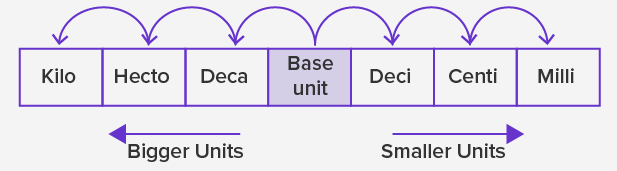# Metric System Worksheets

On this page you will find: a complete list of all of our math worksheets relating to Metric System. Choose a specific addition topic below to view all of our worksheets in that content area. You will find addition lessons, worksheets, homework, and quizzes in each section.

### What is the Metric System?

The metric system is a system of measurement that uses the meter, liter, and gram as base units of length (distance), capacity (volume), and weight (mass) respectively. To measure smaller or larger quantities, we use units derived from the metric units.The given figure shows the arrangement of the metric units, which are smaller or bigger than the base unit. The units to the right of the base unit are smaller than the base unit. As we move to the right, each unit is 10 times smaller or one-tenth of the unit to its left. So, a 'deci' means one-tenth of the base unit, 'centi' is one-tenth of 'deci' or one-hundredth of the base unit and 'milli' is one-tenth of 'centi' or one-thousandth of the base unit. The units to the left of the base unit are bigger than the base unit. As we move to the left, each unit is 10 times greater than the unit to its right. So, a 'deca' means ten times of the base unit, 'hecto' is ten times of 'deca' or hundred times of the base unit and 'killo' is ten times of 'hecto' or thousand times of the base unit.